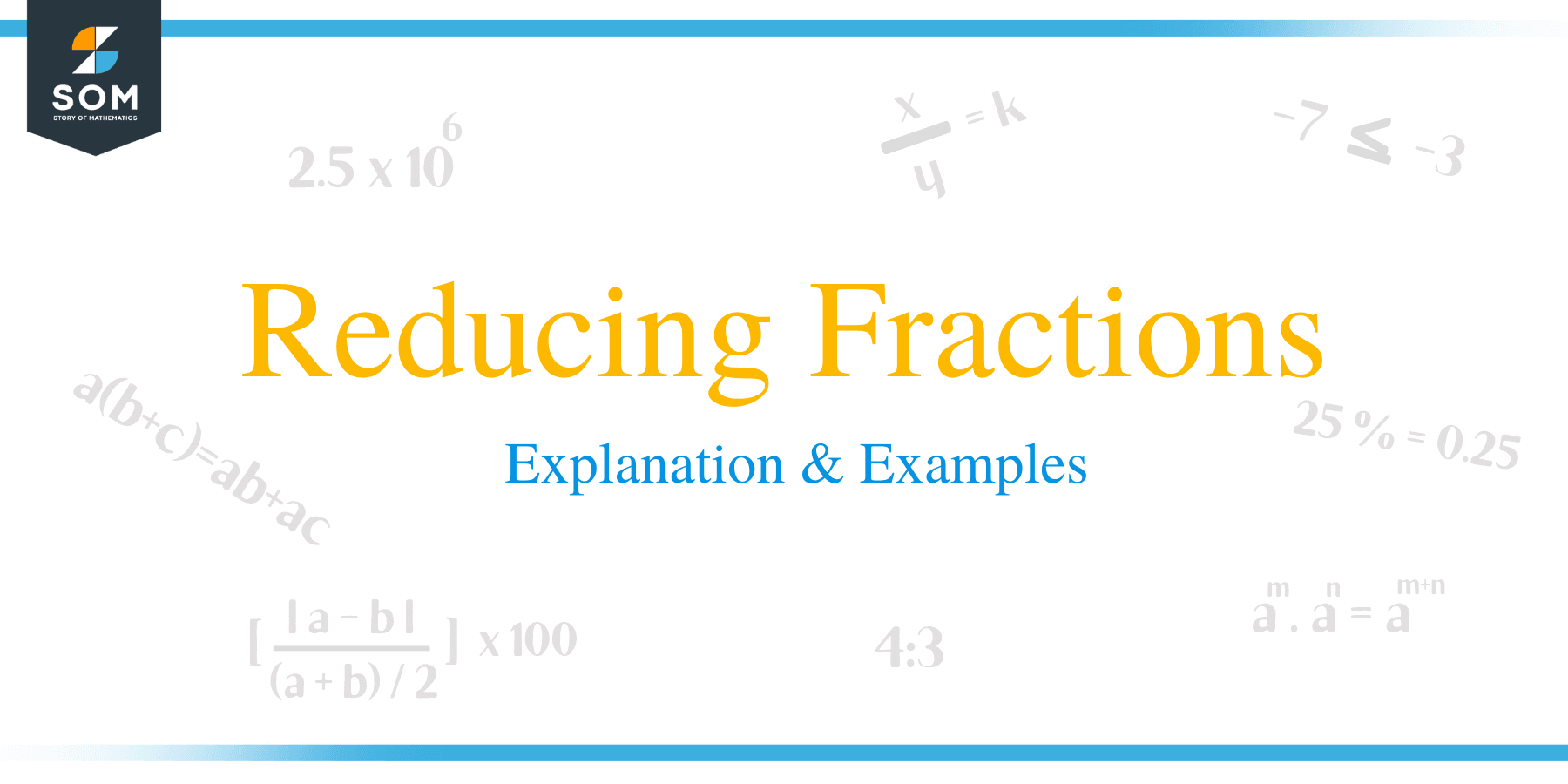# Reducing Fractions – Explanation & Examples

##How to Simplify Fractions?

A fraction can have a numerator and denominator that are composite numbers. There are two methods of how to simplify such a fraction.

Below are the steps on how to reduce a fraction to the lowest possible terms:

• The first step is to identify a common factor of the denominator and numerator.
• The denominator and numerator are both divided by the common factor
• The division operation is repeated until there are no more factors.
• The fraction is said to be simplified if no more factors exitAnother method of simplifying a fraction include:

• Finding the Greatest common factor (GCF) of both the numerator and denominator of a fraction.
• Both the denominator and numerator are divided by the GCF.

Example 1

Simplify the following expression,

3 1/3 ÷ 5/3 – 1/10 of 2 ½ + 7/4

Solution
3 1/3 ÷ 5/3 – 1/10 of 2 ½ + 7/4
= (3 × 3 + 1)/3 ÷ 5/3 – 1/10 of (2 × 2 + 1)/2 + 7/4
= 10/3 ÷ 5/3 – 1/10 of 5/2 + 7/4

= 10/3 × 3/5 – ½ × ½ + 7/4

= 2/1 – ¼ + 7/4
= (2 × 4)/1 × 4) – (1 × 1)/4 × 1) + (7 × 1)/4 × 1)
= 8/4 – ¼ + 7/4

Now the denominators have a common number.
= (8 – 1 + 7)/4
= 14/4
= 7/2

Example 2

Solve and Simplify the answer : 45 of 3/5 ÷ 1 2/3 + 3 of 1/3 – 10

Solution
45 of 3/5 ÷ 1 2/3 + 3 of 1/3 – 10
= 45 of 3/5 ÷ (1 × 3 + 2)/3 + 3 of 1/3 – 10
= 45 of 3/5 ÷ 5/3 + 3 of 1/3 – 10
= 45 × 3/5 ÷ 5/3 + 3 × 1/3 – 10

= 9 × 3 × 3/5 + 3 × 1/3 – 10

= (27 × 3)/5 + 1 – 10
= 81/5 + 1 – 10
= (81 × 1)/(5 × 1) + (1 × 5)/(1 × 5) – (10 × 5)/(1 × 5)
= 81/5 + 5/5 – 50/5

Since the denominators are common for each of the fractions,
= (81 + 5 – 50)/5
= 36/5

= 7 1/5

Example 3

Simplify: {18 + (2 ½ + 4/5)} of 1/1000

Solution
= {18 + (5/2 + 4/5)} of 1/1000
= {18 + ((25 + 8)/10)} of 1/1000
= {18 + 33/10} of 1/1000
= {(180 + 33)/10} of 1/1000
= 213/10 of 1/1000
= 213/10 × 1/1000
= (213 × 1)/(10 × 1000)

= 213/10000
= 0.0213

Example 4

Simplify the following expression:

43 of 1/86 ÷ 1/14 × 2/7 + 9/4 – 1/4

Solution
43 of 1/86 ÷ 1/14 × 2/7 + 9/4 – 1/4
= 43 × 1/86 ÷ 1/14 × 2/7 + 9/4 – 1/4

= 2/1 + 9/4 – 1/4
= (2 × 4)/1 × 4) + (9 × 1)/4 × 1) – (1 × 1)/4 × 1)
= 8/4 + 9/4 – 1/4

Since the denominators are all the same for the fractions,
= (8 + 9 – 1)/4
= 16/4
= 4

Example 5

Simplify: 9/10 ÷ (3/5 + 2 1/10)

Solution
9/10 ÷ (3/5 + 2 1/10)
= 9/10 ÷ (3/5 + 21/10)
= 9/10 ÷ ((6 +21)/10)
= 9/10 ÷ 27/10
= 9/10 × 10/27
= 1/3

Example 6

Simplify: (7 ¼ – 6 1/4) of (2/5 + 3/15)

Solution
(7 ¼ – 6 1/4) of (2/5 + 3/15)
= (29/4 – 25/4) of (2/5 + 3/15)
= ((29 – 25)/4) × ((6 + 3)/15)
= 4/4 × 9/15

Reduce to the fraction to its lowest term

= 1 × 3/5
= 3/5

### Practice Questions

1. A person is carrying $48$ blue balls and $9$ red balls. Which of the following shows the fraction (in simplified form) of the balls that are blue?

2. Alexa’s bookshelf contains $56$ fiction and $14$ nonfiction books. Which of the following shows the fraction (in simplified form) of nonfiction books to the fiction books in Alexa’s shelf?

3. Harold’s shop sells $12$ brands of apparel line and $18$ brands of bags. Which of the following shows the fraction (in simplified form) of apparel brands to bag brands in Harold’s shop?

4. Sam has a piece of wood that is $\dfrac{7}{8}$ meter long. If he needs to cut into pieces of $\dfrac{1}{32}$ meter long each, how many total pieces can Sam cut?

5. Katie has a piece of yarn that is $\dfrac{4}{5}$ yard long. If she needs to cut into pieces of $\dfrac{1}{20}$ yard long each, how many total pieces can Sam cut?